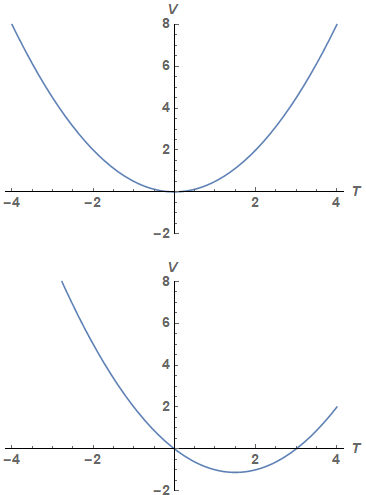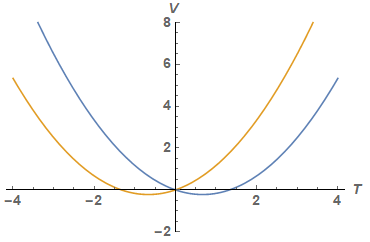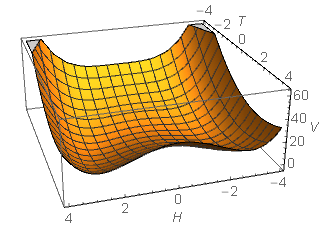# 1. The Vacuum of a Field Theory

© Matt Strassler [April 24, 2022]

Please feel free to point out errors or suggest improvements; this is a dynamic document.

This page is the first of several that together will explain the “Triplet Model”, a very simple method for shifting the W boson mass upward relative to the predictions of the Standard Model.

### Field Theory

A field, in simple math terms, is a function of space and time, F(x,y,z,t), where x,y,z are coordinates of space and t is the coordinate of time.  Often I’ll simplify notation by just writing F(x,t) or F to represent the field; you need to remember it’s a function of all of space and time.

What makes F(x,y,z,t) a field rather than just an arbitrary function that it evolves over time in a relatively simple way.  Field theory is the math that describes how fields change.

Quantum field theory is the math that describes the quantum physics of those fields.  It’s exceedingly complicated and subtle, but fortunately, if we just want to understand the triplet model, we need hardly any of it.

Quantum field theory is a general framework, but any specific example of a quantum field theory, such as the Standard Model of particle physics itself, is defined as having a set of fields and a set of rules that describe how they affect one another.  The rules are encoded in a function of the fields called a “Lagrangian” or a “Hamiltonian”, but we won’t need most of this function.  Instead, we’ll just focus on the “equations of motion”, which are the equations that describe how the fields change. These are analogous to Newton’s second law of motion for ordinary objects (the one that says “F = m a“.)

The only part of the Lagrangian or Hamiltonian we need is the potential-energy density V, often just called “the potential” for short.  As we’ll see in explicit  examples below, V is a function of the fields of the theory (and therefore depends on space and time, since the fields do), and it also depends on various constants.  In the simplest quantum field theories, the equation of motion for a field F takes roughly the following form:

• [(time variation of F)2 – c2(space variation of F)2] = – the variation of V with F

where “c” is the cosmic speed limit, also called the “speed of light.” [If you know calculus, here it is in terms of derivatives

• (d2F/dt2) –  c2(d2F/dx2 + d2F/dy2 + d2F/dz2) = – dV/dF

These really should be partial derivatives, but I’ll be a bit careless here.]

### The Vacuum

The first essential question about any quantum field theory is the nature of its lowest energy configuration, also known as its “ground state” or its “vacuum.” In any universe described by a particular quantum field theory and at a low temperature, you are likely to find most of the universe approximately in this vacuum.

In a vacuum, all fields are usually constant in time and uniform in space.  That’s because any variation with space or time typically requires extra energy… changing things requires more energy than leaving things unchanged… and so a state with varying fields is not the lowest energy configuration.

That means that in a vacuum, the left hand side of the equation of motion, which involves time variation and space variation, is zero, for every field.  Thus, for every field

• in a vacuum, the variation of V with F is zero (or in calculus: dV/dF = 0)

This is necessary (but not sufficient) for an even more general statement:

• in a vacuum state, V (as a function of the fields) must be at a minimum.

This is all very abstract, but specific examples (of which we will have many in what follows) will show you how this works.

Here’s a first, simplest example. Suppose

• V(x,y,z,t) = 1/mT2 [T(x,y,z,t)]2

where mT is a constant and T is a field.  Let’s use our simplifying notation

• V = 1/2 mT2 T2

but you have to keep in your head that T is a function of space and time, while mT is not.

As shown in Fig. 1 (top), this function V = 1/2 mT2 T2 is a parabola, just like y = 1/2 a x2 from grade school algebra. The minimum of y = 1/2 a x2 is at (x,y) = (0,0) — which is also where the slope of the parabola is zero, i.e. where the variation of y with respect to x is zero.  (In calculus terms, dy/dx=0 there.)  For the same reason, V is at its minimum when T(x,y,z,t) = 0 everywhere.  That’s the vacuum of a theory with this choice of potential: T is constant, uniform and zero.Figure 1. Top: V = 1/2 mT2 T2 , for mT = 1; its minimum is at T=0. Bottom: V = 1/2 mT2 T2 – r T, for mT = 1 and r = 3/2; the minimum is at T=+3/2.

Let’s consider another choice: suppose

• V = 1/2 mT2 T2 – r T

where again r and mT2 are constants while T is a field. If T is constant in space and time, this function, shown in Fig. 1 (bottom), is also a parabola, now of this form:

y = 1/2 a x2 – b x = 1/2 a(x – b/a)21/2 b2/a

where in the second expression I completed the square.  Since both terms in the final equation are squares, they are both always positive or zero, and the last one doesn’t depend on x at all.  Therefore the minimum of y occurs when the term in parentheses is as small as it can be — namely zero — and that occurs at x = b/a .

By the identical logic,

• V is at its minimum when T(x,y,z,t) = r/mT2 everywhere.

So in this theory’s vacuum, T is constant, uniform, and non-zero as long as r isn’t zero

The value of T in the vacuum is called its “vacuum expectation value,” or “vev” for short. When r = 0, T’s vev is zero; when r is non-zero, so is T’s vev.  Typical notation for T’s vev is “<T>” or “vT“; we will use both.

### Symmetry

Sometimes a symmetry principle forbids a field from appearing in the potential in an odd number of powers. For example, it might be that (for some reason) the universe has to be symmetric when T is replaced with -T.  In such a universe, terms like T and T3 and T5 could not arise in V.   Why? For instance, the potential

• V = 1/2 mT2 T2 r T

shown in Figure 1 changes when T is replaced with -T; specifically, it becomes

• V = 1/2 mT2 T2 + r TFigure 2: V = 1/2 mT2 T2 – r T (blue) is different from V = 1/2 mT2 T2 + r T (orange) for mT = 1 and r = 2/3, so a universe with +T ⇐⇒ -T symmetry cannot have this potential.

These two different potentials (shown in Figure 2) describe two different universes, unless r=0, in which case they describe just one.  So in any universe that has +T ⇐⇒ -T symmetry, r must be zero, and in fact every term in V must have an even number of powers of T.

Based on what we’ve looked at so far, it might at first seem that <T>, the vev of T, would have to be zero also. But in fact there’s another way to make <T> nonzero.

### Symmetry and the Vacuum

Let’s suppose the universe has the +T ⇐⇒ -T symmetry, and consider the potential

• V = ½ mT2 T2 + ¼ λ T4

where mT2 > 0 and λ > 0 are constants. This potential, like a parabola, looks like a “U”, though it is steeper than a parabola.  Its minimum is at <T>=0 again; the inclusion of λ T4 has had no impact.

But things are different if we consider

• V = – ½ μT2 T2 + ¼ λ T4

where μT2 > 0 is a positive constant, but notice the minus sign in front of it.  That makes the potential look more like a W.  Instead of a minimum at T=0, there is a local maximum there.  The minimum is instead atFigure 3: V = ½ mT2 T2 + ¼ λ T4 (shown for mT = 2 and λ = ¼) looks like a “U” and has a minimum at zero, while V = – ½ μT2 T2 + ¼ λ T4 (shown for μT = 1 and λ = ¼) looks like a “W” and has two minima, at +2 and -2.
• T2 = μT2

(because dV/dT = – μT2 T + λ T3) and thus there are two possible choices for T in the vacuum

• <T> = + μT / λ1/2
• <T> = – μT / λ1/2

(Whether these represent two distinct vacuum states, or just a single state described redundantly, depends on other details of the theory.)

In both of these cases, V has a symmetry — V does not change if you replace T with -T, because only T2 and T4 appear in V.  But this symmetry may not be present in the vacuum.

• when the coefficient in front of the T2 term is positive, then <T>=0; we call this the “unbroken phase“, because in the vacuum <T> = <-T>.
• when the coefficient in front of the T2 term is negative, then <T> is non-zero; we call this the “broken phase“, because in the vacuum <T> and <-T> are not equal.

In the broken phase, something very interesting has happened: even though the universe’s rules have a symmetry, which V respects, the vacuum of the universe does not have this symmetry, because <T> does not respect it.

### Two Fields: First Cartoon of the Triplet Model

One more example we’ll need for the triplet model: two fields H and T, both depending on space and time.  We will imagine that H can only appear in even powers in V, but T is not similarly constrained.  If we take

• V = – ½ μH2 H2 + ¼ λ H4 + ½ mT2 T2 – r T

then the potential has an H piece and separately a T piece, so the logic I’ve used in the previous two cases would apply to H and T separately, with <H> = μH1/2 and <T> = -r/mT2 .

But if I change the r T term to an r H2 T term,

• V = –  ½ μH2 H2 + ¼ λ H4 + ½ mT2 T2r H2 T

now everything’s a bit harder, because in this case the variation of V with respect to T depends on H, and the variation of V with respect to H depends on T. This is illustrated in Figure 4.Figure 4: the potential V = –  ½ μH2 H2 + ¼ λ H4 + ½ mT2 T2 – r H2 T, for particular choices of the constants, showing how its shape depends in a complicated way on H and T.

Still, it’s not that hard. When we try to understand the variation of V with respect to H, we treat T as though it were a constant:

• V = –  ½ H2 + 2 r T) H2 + ¼ λ H4 + ½ mT2 T2

so in the vacuum

• <H> = [μH2 + 2 r <T> ]1/2 / λ1/2 .

Meanwhile when we want the variation of V with respect to T we treat H as a constant

• V = – ½ μH2 H2 + ¼ λ H4 + ½ mT2 T2 – [r H2] T

so

• <T> = r<H>2/mT2

So this gives us two equations that relate the vevs <T> and <H>.  Two equations for two unknowns can be solved.  If we want to, we can solve them exactly:

• <H> =  μH mT / (λ mT2 – 2 r2 )1/2
• <T> = r μH2/ (λ mT2 – 2 r2)

OR if r is small enough, we can solve them approximately

• <H> ≈ μH / λ1/2
• <T> ≈ r μH2/ (λ mT2)

where  “≈” means “almost equals, to the accuracy that we require.” Note that <H> doesn’t depend on r, as long as r is small enough.

Meanwhile, if we instead consider the unbroken phase,

• V = +  ½ mH2 H2 + ¼ λ H4 + ½ mT2 T2 + r H2 T

then the equations imply something different; the minimum of V is at <H>=0 now, and therefore

• <T> =  – r<H>2/mT2 = 0,

even if r is non-zero. In short, T cannot have a non-zero vev unless H does too. This is illustrated in Figure 5.Figure 5: The potential V as a function of H and T, graphed in three dimensions as in Figure 4, but now viewed from “above”. Left: the potential in the broken phase has two minima (grey spots) with H and T both non-zero. If |r/mT2| is small (as shown), then is much bigger than . Right: the potential in the unbroken phase has a single minimum at H=T=0.

The lesson here is that in the broken phase for H, T has a vev as well; in the unbroken phase, neither field does.  For r small enough, it’s H that’s driving everything, with T just following along: when <H> becomes non-zero, it triggers <T> to be slightly non-zero.

This effect is key to the triplet model, where the role of H will be played by the Standard Model Higgs field, and the role of T will be played by the triplet field.

Continued here –>

### 9 thoughts on “1. The Vacuum of a Field Theory”

1.We’ll done. Shook the cobwebs out of my math brain. Too many years working on the project management side of things in engineering and even more years since I really used my Physics degree. That being said, I was able to follow it and deduced that H might very well be the Higgs Field. So, I think you accomplished your goal.

2.You suggest that the ground state and the vacuum state are the same. But this isn’t true for the Simple Harmonic Oscillator, right? The ground state has some energy, but the vacuum state hasn’t. But correct me, if I’m wrong, please.

•Actually, no: “vacuum” does mean “ground state” for the harmonic oscillator. And yes, this state does have zero-point energy.

A field theory’s vacuum has zero-point energy too. (Just because the energy density V is at a minimum doesn’t mean it’s zero!) Formally speaking, though, if you calculate it, you get infinity. It took people quite a while to recognize what this does and doesn’t mean. But this is a very long story… and I won’t cover that here. These issues afflict most field theories, including the Standard Model whether you add a triplet or not.

3.Very clear, interesting and exciting. Thank you.
A correction is required to:
y = 1/2 a x^2 – b x = 1/2 (a x – b)^2 – 1/2 b^2
i.e.:
y = 1/2 a x^2 – b x = 1/2 a (x – b)^2 – 1/2 b^2

•Thanks for catching the error; fixed.

4.“V is at its minimum when T(x,y,z,t) = 0 everywhere.” Is T(x) =0 everywhere true? Doesn’t it imply that T(0) =0 only. Do you need some more proof that T=0 everywhere? What am I missing?

•I withdraw my question!

•5.